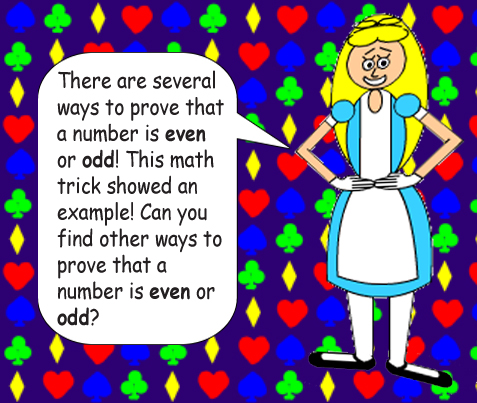# Fraction Frantics

## 1/x = x-1, so (x-1)-1 = x1 = x.If exponents are negative, then you're supposed to divide the base by itself (x number of times) instead of multiply. The base is the number that you're multiplying(or dividing) by itself!

## There are 5 negative 1's as exponents, so since 5 is an odd number, their product is negative. The parentheses are used to multiply them so that you get -1 as the exponential product.Note: Some of the other math tricks show proof that a number is even or odd! Guess which ones they are!